## 14 Most Popular Amazon Coding Interview QuestionsAs the world's largest online marketplace and cloud service provider, Amazon is a dream employer. Landing a job at Amazon is a dream for many software developers.

Did you know that only 2% of people who apply to Amazon are selected? The tech giant has a pretty rigorous hiring process. Amazon's interviewers carefully select coding interview questions to evaluate the applicant's overall abilities.

To succeed at Amazon's coding interviews, you will need a lot of practice, guidance, and the right strategy. As the company receives thousands of resumes each year, you will need to ace your technical interview.

This post will look at the most common Amazon coding questions. I have collected this data from GlassDoor, LeetCode, and CareerCup. You can see solutions to these questions in Grokking the Coding Interview or LeetCode.

### 1. Biggest Island (easy)

Given a 2D array (i.e., a matrix) containing only 1s (land) and 0s (water), find the biggest island in it. Write a function to return the area of the biggest island.
An island is a connected set of 1s (land) and is surrounded by either an edge or 0s (water). Each cell is considered connected to other cells horizontally or vertically (not diagonally).
Example 1
Input: matrix =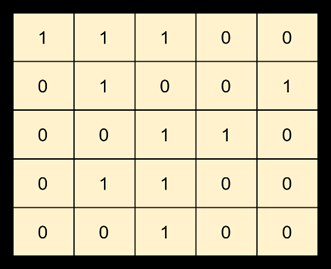Output:5
Explanation: The matrix has three islands. The biggest island has 5 cells.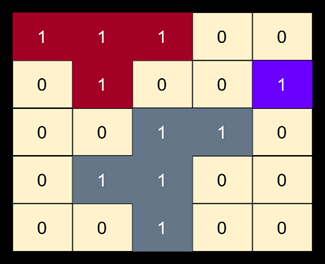### 2. 'K' Closest Points to the Origin (easy)

Given an array of points in a 2D plane, find 'K' closest points to the origin.

Example 1:
Input: points = [[1,2],[1,3]], K = 1
Output: [[1,2]]
Explanation: The Euclidean distance between (1, 2) and the origin is sqrt(5). The Euclidean distance between (1, 3) and the origin is sqrt(10). Since sqrt(5) < sqrt(10), therefore (1, 2) is closer to the origin.

Example 2:
Input: point = [[1, 3], [3, 4], [2, -1]], K = 2
Output: [[1, 3], [2, -1]]Given an array of points in a 2D plane, find 'K' closest points to the origin.

### 3. Right View of a Binary Tree (easy)

Given a binary tree, return an array containing nodes in its right view. The right view of a binary tree is the set of nodes visible when the tree is seen from the right side.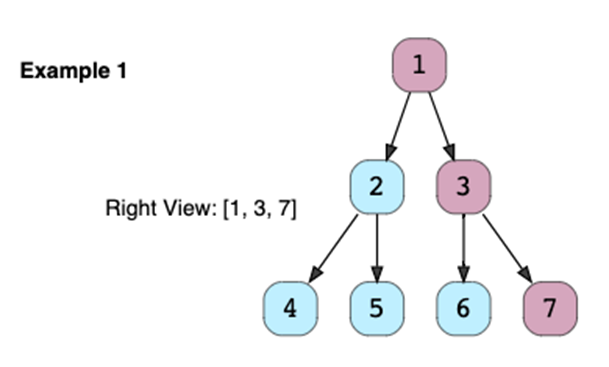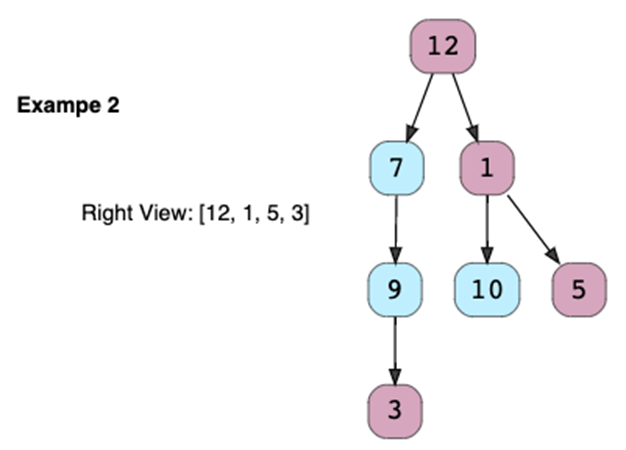### 4. Number of Islands (Medium)

Given a 2D array (i.e., a matrix) containing only 1s (land) and 0s (water), count the number of islands in it.

An island is a connected set of 1s (land) and is surrounded by either an edge or 0s (water). Each cell is considered connected to other cells horizontally or vertically (not diagonally).

Example:
Input: matrix =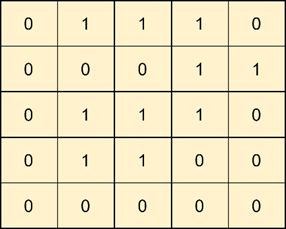Output: 1
Explanation: The matrix has only one island. See the highlighted cells below.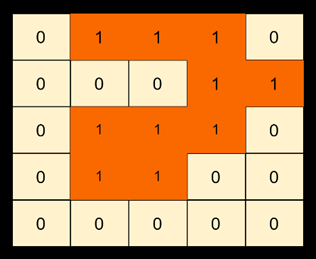### 5. Merge 'K' Sorted Lists (medium)

Given an array of 'K' sorted LinkedLists, merge them into one sorted list.

Example 1:

Input: L1=[2, 6, 8], L2=[3, 6, 7], L3=[1, 3, 4]
Output: [1, 2, 3, 3, 4, 6, 6, 7, 8]

Example 2:
Input: L1=[5, 8, 9], L2=[1, 7]
Output: [1, 5, 7, 8, 9]

There are 'N' tasks, labeled from '0' to 'N-1'. Each task can have some prerequisite tasks which need to be completed before it can be scheduled. Given the number of tasks and a list of prerequisite pairs, find out if it is possible to schedule all the tasks.

Example 1:
Input: Tasks=3, Prerequisites=[0, 1], [1, 2]
Output: true
Explanation: To execute task '1', task '0' needs to finish first. Similarly, task '1' needs to finish before '2' can be scheduled. One possible scheduling of tasks is: [0, 1, 2]

Example 2:
Input: Tasks=3, Prerequisites=[0, 1], [1, 2], [2, 0]
Output: false
Explanation: The tasks have a cyclic dependency, therefore they cannot be scheduled.

Example 3:
Input: Tasks=6, Prerequisites=[2, 5], [0, 5], [0, 4], [1, 4], [3, 2], [1, 3]
Output: true
Explanation: A possible scheduling of tasks is: [0 1 4 3 2 5]

### 7. Median of a Number Stream (medium)

Design a class to calculate the median of a number stream. The class should have the following two methods:

1. insertNum(int num): stores the number in the class
2. findMedian(): returns the median of all numbers inserted in the class

If the count of numbers inserted in the class is even, the median will be the average of the middle two numbers.

Example:
1. insertNum(3)
2. insertNum(1)
3. findMedian() -> output: 2
4. insertNum(5)
5. findMedian() -> output: 3
6. insertNum(4)
7. findMedian() -> output: 3.5

### 8. Merge Intervals (medium)

Given a list of intervals, merge all the overlapping intervals to produce a list that has only mutually exclusive intervals.

Example:
Intervals: [[1,4], [2,5], [7,9]]
Output: [[1,5], [7,9]]
Explanation: Since the first two intervals [1,4] and [2,5] overlap, we merged them into one [1,5].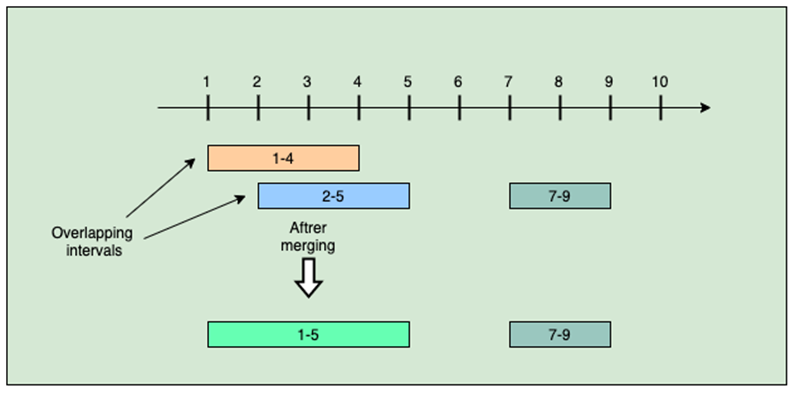### 9. Zigzag Traversal (medium)

Given a binary tree, populate an array to represent its zigzag level order traversal. You should populate the values of all nodes of the first level from left to right, then right to left for the next level, and keep alternating in the same manner for the following levels.

Example: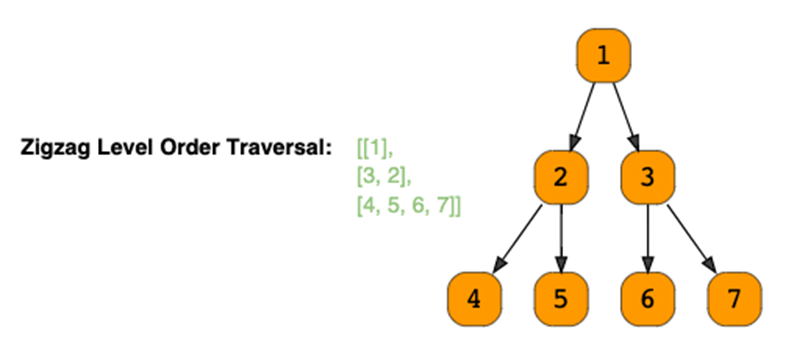### 10. Top 'K' Frequent Numbers (medium)

Given an unsorted array of numbers, find the top 'K' frequently occurring numbers in it.

Example 1:
Input: [1, 3, 5, 12, 11, 12, 11], K = 2
Output: [12, 11]
Explanation: Both '11' and '12' appeared twice.

Example 2:
Input: [5, 12, 11, 3, 11], K = 2
Output: [11, 5] or [11, 12] or [11, 3]
Explanation: Only '11' appeared twice, all other numbers appeared once.

### 11. Minimum Meeting Rooms (hard)

Given a list of intervals representing the start and end time of 'N' meetings, find the minimum number of rooms required to hold all the meetings.

Example 1:
Meetings: [[1,4], [2,5], [7,9]]
Output: 2
Explanation: Since [1,4] and [2,5] overlap, we need two rooms to hold these two meetings. [7,9] can occur in any of the two rooms later.

Example 2:
Meetings: [[4,5], [2,3], [2,4], [3,5]]
Output: 2
Explanation: We will need one room for [2,3] and [3,5], and another room for [2,4] and [4,5].

Here is a visual representation of Example 2: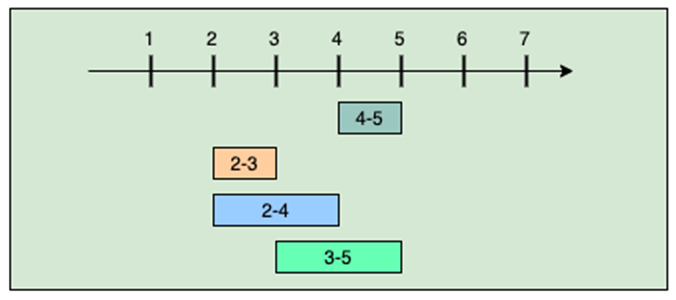### 12. Balanced Parentheses (hard)

For a given number 'N', write a function to generate all combinations of 'N' pairs of balanced parentheses.

Example 1:
Input: N = 2
Output: (()), ()()

Example 2:
Input: N = 3
Output: ((())), (()()), (())(), ()(()), ()()()

### 13. Longest Substring with Distinct Characters (hard)

Given a string, find the length of the longest substring, which has all distinct characters.

Example 1:
Input: String="aabccbb"
Output: 3
Explanation: The longest substring with distinct characters is "abc".

Example 2:
Input: String="abbbb"
Output: 2
Explanation: The longest substring with distinct characters is "ab".

Example 3:
Input: String="abccde"
Output: 3
Explanation: Longest substrings with distinct characters are "abc" & "cde".

### 14. Alien Dictionary (hard)

There is a dictionary containing words from an alien language for which we don't know the ordering of the letters. Write a method to find the correct order of the letters in the alien language. It is given that the input is a valid dictionary and an ordering among its letters exists.

Example 1:
Input: Words: ["ba", "bc", "ac", "cab"]
Output: bac
Explanation: Given that the words are sorted lexicographically by the rules of the alien language, so from the given words we can conclude the following ordering among its characters:

1. From "ba" and "bc", we can conclude that 'a' comes before 'c'.
2. From "bc" and "ac", we can conclude that 'b' comes before 'a'

From the above two points, we can conclude that the correct character order is: "bac"

Example 2:
Input: Words: ["cab", "aaa", "aab"]
Output: cab
Explanation: From the given words we can conclude the following ordering among its characters:

1. From "cab" and "aaa", we can conclude that 'c' comes before 'a'.
2. From "aaa" and "aab", we can conclude that 'a' comes before 'b'

From the above two points, we can conclude that the correct character order is: "cab"

Example 3:
Input: Words: ["ywx", "wz", "xww", "xz", "zyy", "zwz"]
Output: ywxz
Explanation: From the given words we can conclude the following ordering among its characters:

1. From "ywx" and "wz", we can conclude that 'y' comes before 'w'.
2. From "wz" and "xww", we can conclude that 'w' comes before 'x'.
3. From "xww" and "xz", we can conclude that 'w' comes before 'z'
4. From "xz" and "zyy", we can conclude that 'x' comes before 'z'
5. From "zyy" and "zwz", we can conclude that 'y' comes before 'w'

From the above five points, we can conclude that the correct character order is: "ywxz"

## Conclusion

Amazon's coding interviews include LeetCode-type questions. Prepare smartly and focus on the above-mentioned coding problems to increase your chances of success. You can learn more about these questions in Grokking the Coding Interview .